# Clocks - Logical Reasoning (MCQ) Questions

Dear Readers, Welcome to Logical Reasoning Clocks questions and answers with explanation. These Clocks solved examples with shortcuts and tricks will help you learn and practice for your Placement Test and competitive exams like Bank PO, IBPS PO, SBI PO, RRB PO, RBI Assistant, LIC,SSC, MBA - MAT, XAT, CAT, NMAT, UPSC, NET etc.

After practicing these tricky Clocks multiple choice questions, you will be exam ready to deal with any objective type questions.

1)   At what time between 7 and 8 will the hands of a clock be in the same straight line but, not together?
- Published on 15 May 17

a. 5 (5/11) min past 7
b. 5 (4/11) min past 7
c. 5 (3/11) min past 7
d. 5 (2/11) min past 7
 Answer  Explanation ANSWER: 5 (5/11) min past 7 Explanation: When the hands of the clock are in the same straight line but not together, they are 30 minute spaces apart.At 7 o'clock, they are 25 min. spaces apart.Minute hand must gain only 5 min. space.55 min. spaces are gained in 60 min.5 min. spaces would be gained in (60/55) x 5 = 5 (5/11) minRequired time = 5 (5/11) min past 7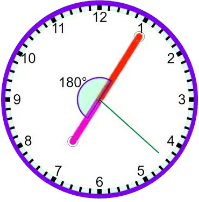2)   At what time between 4 and 4.59 will the hands of a watch point in opposite directions?
- Published on 15 May 17

a. 45 min. past 4
b. 40 min. past 4
c. 50 (4/11) min. past 4
d. 54 (6/11) past 4
 Answer  Explanation ANSWER: 54 (6/11) past 4 Explanation: At 4 o'clock, the hands of the watch are 20 min. spaces apart.To be in opposite directions, they must be 30 min. spaces apart.Minute hand must gain 50 min. spaces.55 min. spaces are gained in 60 min.50 min. spaces are gained in 60/55 x 50 = 54 (6/11) minRequired time = 54 6/11 min past 4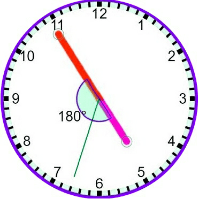3)   The angle between the minute hand and the hour hand of a clock when the time is 8.30, is
- Published on 15 May 17

a. 65
b. 75
c. 85
d. 90
 Answer  Explanation ANSWER: 75 Explanation: At 8 o’ clock, the hour hand is at 240 degrees from the vertical.In 30 minutes,Hour hand = 240 + 30 x (0.5) = 240 + 15 = 255 (The hour hand moves at 0.5 dpm)Minute hand = 30 x (6) = 180 (The minute hand moves at 6 dpm)Difference or angle between the hands = 255 - 180 = 75 degrees.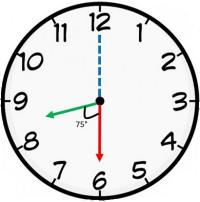4)   At what time, in minutes, between 3 o'clock and 3.25 pm, both the needles of the clock will coincide each other?
- Published on 15 May 17

a. 13 (4/11)
b. 14 (4/11)
c. 15 (4/11)
d. 16 (4/11)
 Answer  Explanation ANSWER: 16 (4/11) Explanation: At 3 o'clock, the minute hand is 15 min. spaces apart from the hour hand.To be coincident, it must gain 15 min. spaces.55 min. are gained in 60 min15 min. spaces are gained in 60/55 x 15 = 16 4/11 minThe hands are co-incident at 16 (4/11) past 3.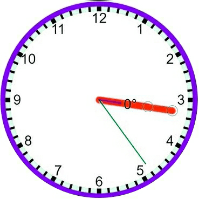5)   A clock is set right at 5 am the clock loses 16 min in 24 hours. What will be the right time when the clock indicates on 3 pm the 8th day?
- Published on 08 May 17

a. 4 pm
b. 5 pm
c. 6 pm
d. 7 pm
 Answer  Explanation ANSWER: 5 pm Explanation: Time from 5 am On a day to 3 pm on 8th day = 178 hours Now 23 hrs 44 min. of this clock = 24 hours of correct clock 365/15 hrs of this clock = 24 hours of correct clock 178 hrs of this clock = (24 × 15/356 × 89) hrs of correct clock= 180 hrs of correct clock. So, the correct time is 5 pm.

6)   How much does a watch gain or lose per day, if its hands coincide every 64 minutes?
- Published on 08 May 17

a. Gain 92 min
b. Lose 9 min
c. Lose 34 (5/11) min
d. Lose 32 (8/11) min
 Answer  Explanation ANSWER: Lose 32 (8/11) min Explanation: 55 min. spaces are covered in 60 min.60 min. spaces are covered in (60/55 × 60) min = 65 5/11 min Loss in 64 min = (65 5/11 - 64) = 16/11 minLoss in 24 hrs= (16/11 × 1/64 × 24 × 60) min = 32 (8/11) min.

7)   At what time between 7.02 and 8.02 will the hands of a watch be in the straight line but no together?
- Published on 08 May 17

a. 5 (3/11) min past 7
b. 5 (5/11) min past 7
c. 5 (3/11) min past 7
d. 43 min past 7
 Answer  Explanation ANSWER: 5 (5/11) min past 7 Explanation: When the hands of the clock are in the same straight line but not together, they are 30 minute spaces apart.At 7 o'clock, they are 25 min. spaces apart. Minute hand must gain only 5 min. space55 min. spaces are gained in 60 min.5 min. spaces are gained in (60/55 × 5) min = 5 (5/11) min. Required time = 5 (5/11) min. past 7.

8)   At what time between 3 & 4 will the hands of a watch point in opposite directions?
- Published on 03 May 17

a. 43 min past 3
b. 42 (5/11) min past 3
c. 49 (1/11) min past 3
d. 50 min past 3
 Answer  Explanation ANSWER: 49 (1/11) min past 3 Explanation: At 3 o'clock, the hands of the watch are 15 min. space apart.To be in opposite directions, they must be 30 min. spaces apart. Minute hand must gain 45 min. space (15 + 30 min)55 min spaces are gained in 60 min. 45 min. spaces would be gained in (60/55 × 45) min. Required time = 49 1/11 min. past 3.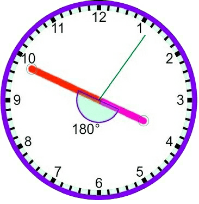9)   How many times do the hands of a clock coincide in a day?
- Published on 25 Apr 17

a. 11
b. 22
c. 12
d. 24

Explanation:
The hands of a clock coincide 11 times in every 12 hours (Since between 11 and 1, they coincide only once, i.e., at 12 o'clock).

 AM 12 1.05 2.11 3.16 4.22 5.27 6.33 7.38 8.44 9.49 10.55 PM 12 1.05 2.11 3.16 4.22 5.27 6.33 7.38 8.44 9.49 10.55
The hands overlap about every 65 minutes, not every 60 minutes.
Therefore, The hands coincide 22 times in a day.

10)   When the time in the clock is 7.20 pm, then the angle between the hands of the clock is
- Published on 25 Apr 17

a. 80
b. 90
c. 100
d. 110
 Answer  Explanation ANSWER: 100 Explanation: At 7 o’ clock, the hour hand is at 210 degrees from the vertical.In 20 minutes,Hour hand = 210 + 20 x (0.5) = 220 (The hour hand moves at 0.5 dpm)Minute hand = 20 x (6) = 120 (The minute hand moves at 6 dpm)Difference or angle between the hands = 220 - 120 = 100 degree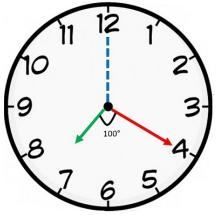1 2